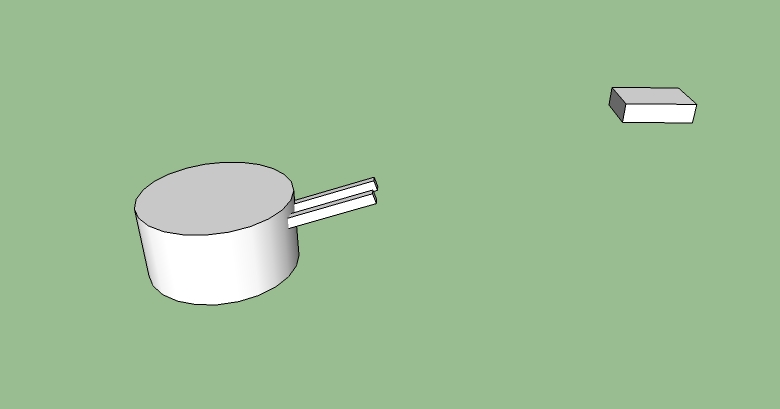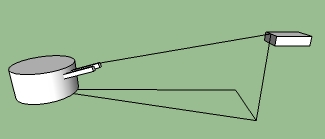CS 420 Game Engineering 3D
Final Practice Problems
1. You want to point your camera at an object.  You want the camera be as close as possible to "right side up", given that it is pointed at the object.  That is, you want the camera's up vector to be as close as possible to the world up vector, given that the camera is pointed directly at the object.  If the object has the position po = [pox, poy, poz], and the camera has the position pc = [pcx, pcy, pcz], give the rotational matrix for the camera.

First, we will find the facing vector of the camera, fv.  This is the easiest one -- just the normalized vector from pc to po.

fvpo - pc / || po - pc ||

Next, we will find the left vector (assuming a right-handed coordinate system).  First, we know that the left vector needs to be perpendicular to the facing vector -- but there is an entire plane, an infinite number of vectors, perpendicular to the facing vector.  Since we want the up vector to be as close as possible to vertical, that means that we want the left vector to be as close as possible to horizontal -- in fact, we want the left vector to be parallel to the ground plane, and hence perpendicular to the global up vector.  Since we now have two vectors that are both perpendicular to the vector that we want, we can solve it with a simple cross product:

lv = [0, 1, 0] × fv / || [0, 1, 0] × fv ||

Note that the order is important here -- if we did the cross product in the opposite order, we would get the wrong sign for our left vector.  If we were calculating the matrix for a model, this would turn the model inside out.

There is one more wrinkle to finding the left vector -- what happens when the camera needs to point straight up?  That is, what happens when the facing vector is [0, 1, 0]?  (or [0, -1, 0], for that matter?)  Any vector cross itself is the zero vector, and we get a division-by-zero error.  Of course, if the camera is pointing straight up (or straight down), then it doesn't matter what left vector we choose, as long as it is perpendicular to the facing vector.  Any one will do, so if fv = [0, 1, 0] or fv = [0, -1, 0], we'll set  lv = [1, 0, 0].

Once we have fv and lv, finding the up vector is easy, and can be done with a cross product.  Be careful abou the order!

uv = fv  × lv

Since fv and lv are mutually perpendicular unit vectors, we do not need to normalize uv

Our rotational matrix is composed of these three vectors:

 lv [ uv ] fv

2. You want to aim a turret.  The turret consists of two objects:  The "base", which can rotate around the y axis, and the barrel, which can be raised or lowered:The barrel is attached to the turret (so that it moves when the turret moves).  The barrel is attached at an offset of [0,0,0] in turret space (so the origin of the barrel is the same as the origin of the turret).  Assume that if the rotational matrix of the barrel (in turret space) is the identity matrix I, the barrel points straight down the z-axis of the turret.

You wish to rotate the turret and raise the barrel so that it points at an object at position p = [px, py, px].   Show how to create the rotational matrix for both the turret (in world space) and the barrel (in turret space).   Feel free to define "local variables" in your answer (such as, let x = || p1 × p2||)

We'll first assume that the turret is at the origin, level with the ground plane.  We'll start with the turret.  The up vector is easy:  [0, 1, 0].  The facing vector needs to point directly at the object, projected into the xz plane.  Thus, the facing vecor must be  [px, 0, pz] / || [px, 0, pz] ||.  The left vector is just the up vector cross the facing vector:  [0, 1, 0] × ([px, 0, pz] / || [px, 0, pz] ||).

Next, we can do the barrel.  The left vector is easy -- just [1, 0, 0].  The facing vector is a little trickier.  We know that the x component of the facing vector should be 0.  We also know that the slope of the line must be:  py / sqrt(px2 + pz2)From that we get a facing vector of [0,  py, sqrt(px2 + pz2)] / || [0,  py, sqrt(px2 + pz2)] ||

The Up vector of the barrel is just the facing vector cross the left vector.

So:

Turret:

Left: [0, 1, 0] × ([px, 0, px] / || [px, 0, px] ||)
Up: [0, 1, 0]
Facing: ([px, 0, px] / || [px, 0, px] ||)

Barrel
Left: [1, 0, 0]
Up: [0,  py, sqrt(px2 + pz2)] / || [0,  py, sqrt(px2 + pz2)] || × [1, 0, 0]
Facing: [0,  py, sqrt(px2 + pz2)] / || [0,  py, sqrt(px2 + pz2)] ||

3. You are representing planets in a solar system using the following scheme:
• The position of the sun us given a s a 3-Vector in world space possun
• The location of planet p1 is given as a scalar distance from the sun dp1 and orientation (quaternion) qp1 which describes how much we need to rotate the vector [0, 0, dp1] so that it points from the sun to planet p1
• Likewise, the location of planet p2 is given as a scalar distance from the sun dp2 and orientation (quaternion) qp2 which describes how much we need to rotate the vector [0, 0, dp2] so that it points from the sun to planet p2
• The location of moon m1 is given as a scalar distance from the planet p1, dm1 and orientation (quaternion) qm1 which describes how much we need to rotate the vector [0, 0, dm1] so that it points from the planet p11
• The location of moon m2 is given as a scalar distance from the planet p2, dm2 and orientation (quaternion) qm2 which describes how much we need to rotate the vector [0, 0, dm2] so that it points from the planet p22
Give an equation that describes the scalar distance between moon m1 and moon m2 as a function of possun, dp1, qp1,dp2, qp2, dm1, qm1,dm2 and qm2,
Position of Moon 1 in world space: qm1 * [0, 0, dm1] + qp1 * [0, 0, dp1] + ps
Position of Moon 2 in world space:  qm2 * [0, 0, dm2] + qp2 * [0, 0, dp2] + ps

Distance between Moon1 and Moon2: || qm1 * [0, 0, dm1] + qp1 * [0, 0, dp1] + ps - (qm2 * [0, 0, dm2] + qp2 * [0, 0, dp2] + ps) ||
4. What is the output of the following fragment of C++ code:
```int &weird(int &x)
{
return ++x;
}

int main()
{
int x = 1;
int &y = weird(x);
printf("x = %d, y = &d \n", x, y);
y++;
printf("x = %d, y - %d \n", x, y);
}
```
Ouput:
```x = 2, y = 2
x = 3, y = 3
```
Bonus question:  If we change the ++x to a x++, then the code will no longer compile.  Why not?
When we return ++x, we are incrementing x, and then returning x.  Since we are returning a reference to x, we just return a pointer to x after its value has been incremented.  What happens if we try to return x++?   Let's take a look at what happens when we try to return x++ from a function that is returning a value, and not a reference.  First, we store the old value of x in a temporary variable, then increment x, and then return the temporary.  If we are returning a reference, then we can't return a pointer to that temporary value -- the temporary ceases to exist after the function ends, and dereferencing it would cause a bus error.  C++ does a little more compile-time checking on references than it does with raw pointers.  For reference, the actual compiler error is:

error: invalid initialization of non-const reference type 'int &' from a temporary of type 'int'

5. What is the output of the following fragment of lua code:
1. ```MyTable = { = 2,  = 2,  = 1,  = 1}
s1 = 0
s2 = 0
for k,v in pairs(MyTable) do
s1 = s1 + k
s2 = s2 + v
end
print(s1,s2)
```
Output:
```15      6
```
2. ```x = 2
y = 3
function twist()
local x = 7
x,y = y, x
print(x,y)
end
twist()
print(x,y)
```
Output
```3        7
2        7
```
3. ```ft = {}
ft  = function(x, y) return y,x end
ft  = function(x, y, z) return z, y, x end
a, b, c = ft(1,2,3)
d, e, f = ft(4,5,6)
print(a, b, c)
print(d, e, f)
```
Output:
```2    1    nil
6    5    4
```